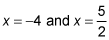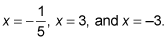##### Algebra II For DummiesFactoring by grouping terms is a great method to use to rewrite a quadratic equation so that you can use the multiplication property of zero and find all the solutions.

The main idea behind factoring by grouping is to arrange the terms into smaller groupings that have a common factor. You go to little groupings because you can't find a greatest common factor for all the terms; however, by taking two terms at a time, you can find something to divide them by.

For example, look at the quadratic equation

2x2 + 8x – 5x – 20 = 0,

which has four terms. (Although you could combine the two middle terms on the left, in this case, leave them as is for the sake of the grouping process.)

The four terms in the equation don't share a greatest common factor. You can divide the first, second, and fourth terms evenly by 2, but the third term doesn't comply. The first three terms all have a factor of x, but the last term doesn't. So, you group the first two terms together and take out their common factor, 2x. The last two terms have a common factor of –5. The factored form, therefore, is

2x(x + 4) – 5(x + 4) = 0.

The new, factored form has two terms. Each of the terms has an (x + 4) factor, so you can divide that factor out of each term. When you divide the first term, you have 2x left. When you divide the second term, you have –5 left. Your new factored form is

(x + 4)(2x – 5) = 0.

Now you can set each factor equal to zero to getKeep in mind that factoring by grouping works only when you can create a new form of the quadratic equation that has fewer terms and a common factor. If the factor (x + 4) hadn't shown up in both of the factored terms in this example, you would've gone in a different direction.

Solving quadratic equations by grouping and factoring is even more important when the exponents in the equations get larger. For example, the equation

5x3 + x2 – 45x – 9 = 0

is a third-degree equation (the highest power on any of the variables is 3), so it has the potential for three different solutions. You can't find a factor common to all four terms, so you group the first two terms, factor out x2, group the last two terms, and factor out –9. The factored equation is

x2(5x + 1) – 9(5x + 1) = 0

The common factor of the two terms in the new equation is (5x + 1), so you divide it out of the two terms to get

(5x + 1)(x2 – 9) = 0

The second factor is the difference of squares, so you can rewrite the equation as

(5x + 1)(x – 3)(x + 3) = 0

The three solutions are